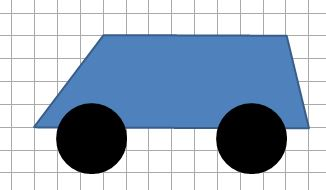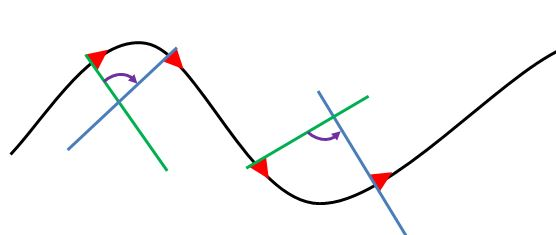# Making a Simple Car

This is to show the practical use of rotate.

## The Design

The car will consist of a trapezoid for the body, four cylinders for wheels. The car will be animated to follow a path an remain parallel to it. The wheels will rotate and be given a texture to show that they are rotating. For simplicity all wheels will remain parallel to the car and their rotation will not be matched to the forward speed of the car. The car will move forward by following a sequence of points on the path. The path will be a curved line with large turning radius and will be shown in the scene.### Car body

The car body will be an extrusion of the trapezium formed from the trapezium given by the points (-4, 2), (4, 2), (5, -2), (-7, -2) in the direction of the positive x axis from (0, 0, 0) to (0, 0, 4)

var bodyMaterial = new BABYLON.StandardMaterial("body_mat", scene);bodyMaterial.diffuseColor = new BABYLON.Color3(1.0, 0.25, 0.25);bodyMaterial.backFaceCulling = false;
var side = [new BABYLON.Vector3(-4, 2, -2),			new BABYLON.Vector3(4, 2, -2),			new BABYLON.Vector3(5, -2, -2),			new BABYLON.Vector3(-7, -2, -2)];
side.push(side);	//close trapezium
var extrudePath = [new BABYLON.Vector3(0, 0, 0), 	new BABYLON.Vector3(0, 0, 4)];
var carBody = BABYLON.MeshBuilder.ExtrudeShape("body", {shape: side, path: extrudePath, cap : BABYLON.Mesh.CAP_ALL}, scene);carBody.material = bodyMaterial;

PG: Car Body

### Wheel

The wheel is made from a cylinder using MeshBuilder as this allows different textures and colours to be applied to the flat and curved surfaces of the cylinder.

var wheelMaterial = new BABYLON.StandardMaterial("wheel_mat", scene);var wheelTexture = new BABYLON.Texture("https://i.imgur.com/ZUWbT6L.png", scene);wheelMaterial.diffuseTexture = wheelTexture;
//Set color for wheel tread as blackvar faceColors=[];faceColors = new BABYLON.Color3(0,0,0);
//set texture for flat face of wheelvar faceUV =[];faceUV = new BABYLON.Vector4(0,0,1,1);faceUV = new BABYLON.Vector4(0,0,1,1);
var wheel = BABYLON.MeshBuilder.CreateCylinder("wheel", {diameter: 3, height: 1, tessellation: 24, faceColors:faceColors, faceUV:faceUV}, scene);wheel.material = wheelMaterial;
//rotate wheel so tread in xz planewheel.rotation.x = Math.PI/2;

PG: Wheels

### Attaching the Wheels to the Car

As the car moves forward so will the wheels, this can be achieved by making the car the parent of the wheels.

As the wheels need to first rotate around the x axis to orientate the cylinder correctly and then rotate about another axis the rotate method will be used instead of rotation.

var wheelFI = BABYLON.MeshBuilder.CreateCylinder("wheelFI", {diameter: 3, height: 1, tessellation: 24, faceColors:faceColors, faceUV:faceUV}, scene);wheelFI.material = wheelMaterial;
wheelFI.rotate(BABYLON.Axis.X, Math.PI/2, BABYLON.Space.WORLD);wheelFI.parent = carBody;
var wheelFO = wheelFI.createInstance("FO");wheelFO.parent = carBody;wheelFO.position = new BABYLON.Vector3(-4.5, -2, 2.8);
var wheelRI = wheelFI.createInstance("RI");wheelRI.parent = carBody;wheelRI.position = new BABYLON.Vector3(2.5, -2, -2.8);
var wheelRO = wheelFI.createInstance("RO");wheelRO.parent = carBody;wheelRO.position = new BABYLON.Vector3(2.5, -2, 2.8);
wheelFI.position = new BABYLON.Vector3(-4.5, -2, -2.8);

PG: Attach Wheels

### Rotate Wheels

The animation is achieved using scene.registerAfterRender and small rotations within the render loop.

Note: when using rotate and translate scene.registerAfterRender is preferable to scene.registerBeforeRender as the calculations will take place after the world matrix has been computed for each mesh.

scene.registerAfterRender(function() {	 wheelFI.rotate(BABYLON.Axis.Z, Math.PI/64, BABYLON.Space.WORLD);	 wheelFO.rotate(BABYLON.Axis.Z, Math.PI/64, BABYLON.Space.WORLD);	 wheelRI.rotate(BABYLON.Axis.Z, Math.PI/64, BABYLON.Space.WORLD);	 wheelRO.rotate(BABYLON.Axis.Z, Math.PI/64, BABYLON.Space.WORLD);
});

PG: Rotate Wheels

### Path

To draw a path a sequence of points are needed. Starting with a circle of radius r in the xz plane with centre (0, 0, 0) calculate points around the circle and vary r slightly and smoothly on the way round to give a less regular path. This is achieved by adding to the radius, at each angle, a fraction of r times the sine of a multiple of the angle turned. In addition a ground is added.

Since on creation the side of the car is perpendicuar to the z axis the base circle will start with theta = 0 and x = r * sin(theta) and z = r * cos(theta). This means that when the car can be positioned tangental to the circle by setting z = r.

Since the circle has been given a perturbation some small rotation of the car will be necessary for it to be truly tangential.

var points = [];var n = 50; // number of points - more points the slower the carvar r = 50; //radiusfor (let i = 0; i < n + 1; i++) {	points.push( new BABYLON.Vector3((r + (r/5)*Math.sin(8*i*Math.PI/n))* Math.cos(2*i*Math.PI/n), 0, (r + (r/10)*Math.sin(6*i*Math.PI/n)) * Math.sin(2*i*Math.PI/n)));}
var track = BABYLON.MeshBuilder.CreateLines('track', {points: points}, scene);track.color = new BABYLON.Color3(0, 0, 0);
var ground = BABYLON.MeshBuilder.CreateGround("ground", {width: 3*r, height: 3*r}, scene);

PG: The Path

### Following the Path

The car will be moved forward using the sequence of points calculated for the path. As it moves round the path the car side should remain tangential to the path and the wheels should rotate about an axis that is normal to the path. Since the normals to the path need to be calculated for the wheel axes it will be the normals that will be used to rotate the car. The angle to be rotated at any point can be found from the angle between the previous normal and the current one. This angle can be found by using the dot product of the two normal vectors. However the dot product only gives the amount of rotation not the direction.

Determining the normals at any point is achieved by forming the Path3D object from the array of points defining the path and extracting the normals which are unit vectors.

var path3d = new BABYLON.Path3D(points);var normals = path3d.getNormals();

As can be seen from the diagram below the direction that the normal changes varies from clockwise to anti-clockwise depending whether the path is a convex or concave curve.Both normals lie in the xz plane and so the cross product of the two normals will lie in the y axis and so the direction of turn can be determined from the sign of the y component of the cross product.

theta = Math.acos(BABYLON.Vector3.Dot(normals[i],normals[i+1]));  //amount of turnvar dir = BABYLON.Vector3.Cross(normals[i],normals[i+1]).y; //enables direction of turn to be found depending if +ve or -vevar dir = dir/Math.abs(dir); //dir takes value 1 or -1carBody.rotate(BABYLON.Axis.Y, dir * theta, BABYLON.Space.WORLD);

### Placing the Car

To stand on the ground the car will need to be lifted. To be set on the path it will be moved to the point (0, r, 4). Its current normal is along the z axis so determine the turn necessary from

var theta = Math.acos(BABYLON.Vector3.Dot(BABYLON.Axis.Z,normals));carBody.rotate(BABYLON.Axis.Y, theta, BABYLON.Space.WORLD);

### Animating the Car

The animation is achieved using scene.registerAfterRender. Within this render loop: the car is moved forward from point to point; it is rotated tangential to the path at the current point; the wheels are now rotated a small amount about the normal at the current point.

var i=0;scene.registerAfterRender(function() { carBody.position.x = points[i].x; carBody.position.z = points[i].z; wheelFI.rotate(normals[i], Math.PI/32, BABYLON.Space.WORLD); wheelFO.rotate(normals[i], Math.PI/32, BABYLON.Space.WORLD); wheelRI.rotate(normals[i], Math.PI/32, BABYLON.Space.WORLD); wheelRO.rotate(normals[i], Math.PI/32, BABYLON.Space.WORLD);
theta = Math.acos(BABYLON.Vector3.Dot(normals[i],normals[i+1])); var dir = BABYLON.Vector3.Cross(normals[i],normals[i+1]).y; var dir = dir/Math.abs(dir); carBody.rotate(BABYLON.Axis.Y, dir * theta, BABYLON.Space.WORLD);
i = (i + 1) % (n-1);	//continuous looping});

PG: Car Travels The Path

## Correction

After allowing the finalised playground of the car to run for a while it can be seen that the rotating of the car becomes askew. This is due to rounding errors in the floating point (probably).

The initial rotation quaternion is stored in startRotation and is now applied to the car whenever it starts a new loop.

if(i == 0) {	carBody.rotationQuaternion = startRotation;}

PG: Car Following a Path